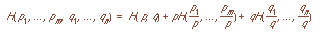# Homework #1

UC Davis Students: Due April 14, 1997 at 11:59PM
NTU Students: Due April 21, 1997 at 11:59PM

1. (40 points) The entropy function H(p1, ..., pn) must satisfy several properties, including the following (for which we assume p1 + ... + pn = 1):
1. H(p1, ..., pn) is a maximum when p1 = ... = pn = 1/n.
2. For any permutation ¼ (Greek letter pi) on (1, 2, ..., n), H(p1, ..., pn) = H(p¼(1), ..., p¼(n)). In other words, H must be a symmetric function of the arguments p1, ..., pn.
3. H(p1, ..., pn) >= 0 and equals 0 only when one of the pi = 1.
4. H(p1, ..., pn, 0) = H(p1, ..., pn).
5.6. If m and n are positive integers, then7. Let p = p1 + ... + pm and q = q1 + ... + qn, where each pi and qj are non-negative; then, if p and q are positive, with p + q = 1, we must havePlease prove that the function(where the sum is over those k for which pk > 0) meets these conditions.

2. (20 points)Let X be an integer variable represented with 32 bits. Suppose that the probability is 1/2 that X lies in the range [0, 28-1] with all such values being equally likely, and 1/2 that X lies in the range [28, 232-1], with all such values being equally likely. Please compute H(X).

3. (10 points)Consider the following ciphertests:

VZXYW
ACRYZ
QWERT
ZZZZZ
Which of these ciphertexts could result from enciphering five-letter words of English using:
1. A substitution cipher, where each letter is replaced with some other letter, but the letters are not necessarily shifted as in the Caesar cipher (thus A could be replaced with X, B with U, etc.)?
2. Any transposition cipher?

4. (10 points) Let n be a composite number. Please show there are integers x and y such that xy mod n = 0, but neither x nor y is 0 mod n. (This proves the cancellation law does not hold over the integers mod n with multiplication.)

5. (40 points) The following was enciphered with a Vigènere cipher. Please break it.
TSMVM MPPCW CZUGX HPECP RFAUE IOBQW PPIMS
FXIPC TSQPK SZNUL OPACR DDPKT SLVFW ELTKR
GHIZS FNIDF ARMUE NOSKR GDIPH WSGVL EDMCM
SMWKP IYOJS TLVFA HPBJI RAQIW HLDGA IYOUX

6. (20 points) Please determine the unicity distance of ciphers based on the affine transformation
f(x) = (ax + b) mod 26
assuming the keys a and b both generate complete sets of residues and that all such keys are equally likely.

7. (10 points) The ciphertext TKCXJ YMRES ATKMZ came from a message enciphered using the Playfair cipher with the following key:
I E N S B
R Y T O K
C P G A H
D F L M Q
U V W X Z

8. (20 points) If you are given two Playfair ciphers P1 and P2 which are applied one after the other to a string of text T, i.e., P2(P1(T)), do you have a cipher which is more secure than using only P1 or only P2?

9. (10 points) Please compute the following:
1. 212334565789 mod 4;
2. 6512 mod 15

10. (15 points) Solve the following problems for x in the range [0, n-1]:
1. 1234x mod 10001 = 1
2. 23x mod 57 = 1
3. 17x mod 100 = 10.

11. (5 points) Find all solutions to the equation 15x mod 25 = 10 in the range [0, 24].

### Extra Credit

1. Given the additional requirement that H(p1, ..., pn) is a continuous function of its arguments, prove that the only function meeting all these requirements iswith l any positive constant and where the sum is over those k for which pk > 0.

2. Write a program to break a Caesar cipher automatically, assuming the plaintext is English. You may use any reasonable model of English (either single letter counts, n-gram counts, or a Markov model).

You can get this document in Postscript, ASCII text, or Framemaker version 5.1.Send email to cs253@csif.cs.ucdavis.edu.

Department of Computer Science
University of California at Davis
Davis, CA 95616-8562